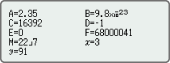My Cart

Mini Cart

Casio fx-570EX | ClassWiz | Non programmable | scientific calculator (FX570EX)
87

Casio fx-570EX | ClassWiz | Non programmable | scientific calculator (FX570EX)

RM87.00

Number of Functions : 552

GCSE/AS/A Level

List based STAT-data editor

10 + 2 digits

AAA × 1 (R03)

- +

Extended Warranty Programme

Availability:In stock
SKU FX570EX
Brand CASIO
Warranty Period: 1 Year
Delivery: Nationwide (T&C Apply)
Details
Features
Intuitive, easy-to-learn icon displayThe use of icons on the menu screen improves viewability. Selection of the desired function is quick and easy.

Natural Textbook DisplayInput and display fractions, powers, logarithms, roots, and other mathematical formulas and symbols just as they appear in textbooks.

List display function for thorough, speedy learningVariables and statistical calculation results stored in memory can be displayed in lists. There’s no need to recall and confirm individual values as with previous models.

Easy-to-understand English messages and interactive formatNearly all English words are displayed on the screen in unabbreviated form. Interactive menu display realizes more intuitive operation.

High computational competence for performing even advanced mathematicsClassWiz contains calculation functions that support even advanced mathematical operations, including spreadsheet calculations, 4 × 4 matrix calculations, calculation of simultaneous equations with four unknowns and quartic equations, and advanced statistical distribution calculations.

Online Visualization Service Using QR CodeGenerate QR codes of equations input into the calculator by a simple operation. Graphs and other graphics can be displayed on smartphone or tablet screens.

A comprehensive set of advanced functions unique to CASIO scientific calculatorsUseful for learning statistics. Up to 5 columns × 45 rows (maximum of 170 data items)
Matrix calculationsPerform calculations with matrices of up to 4 rows and 4 columns.
Vector calculationsPerform calculations using up to four third-order vectors stored in memory.
Multiline displayFormulas are automatically wrapped to multiple lines. *
*In linear input mode
Integration calculationsPerform integration calculations in advanced mathematics.
Differential calculationsPerform differential calculations in advanced mathematics.
Equation calculationsCalculation of simultaneous equations in 2 to 4 unknowns and high-degree equations of second to fourth degree
Inequality calculationsSolve second-degree to fourth-degree inequalities.Perform calculations involving normal distributions, binomial distributions, and Poisson distributions.
Ratio calculationsPerform ratio calculations such as A:B=X:D.
Metric conversionsSelect units from the Metric Conversion Command List.
Scientific constantsSelect scientific constants from the Scientific Constant Table.
Variables listOne-touch list display
Statistics listDisplays six rows at once.
Digit separatorSeparation every three digits makes even large numbers easy to read.
Engineering symbolsPerform engineering calculations that include symbols such as k, M, m, and n.

Specifications

• Non Programmable

•GCSE/AS/A LevelSuitable for Cambridge International Examinations GCSE, AS & A Level.

•Number of Functions : 552

•Natural textbook displayDisplay expression same as textbook.

•AAA × 1 (R03)

•10 + 2 digits10-digit mantissa + 2-digit exponential display.

•Dot matrix displayHigh-resolution screen provides beautiful looking graphs every time.

•Icon menuSpecify the operation you want to perform by selecting an icon or inputting a number.

•Multi-replayQuick and easy recall of previously executed formulas for editing and re-execution.

•Plastic keysDesigned and engineered for easy operation.

•List based STAT-data editorViewing and editing of input data in list format, showing data groups (x-data, y-data, frequency) and surrounding data.

• Basic StatisticsStandard statistics functions such as Mean, SUM, Standard Deviation, and Regression

• Ratio

• Basic Mathematical FunctionsTrigonometric, Exponential logarithmic, etc.

• Table GenerationYou can create a numerical table based on that expression by registering a function expression. In addition, graph functions can be represented by graphs.

• Intermediate StatisticsMore statistical functions such as Paired-variable statistical calculation, Quartiles and List display functions for speedy and thorough learning .

• Scientific ConstantsYou can use constant symbols used in physics, science classes.

• Vector

• Advanced StatisticsSupport high level statistics by 7 kinds of advanced distribution calculation.

• Inequality

• AAA-size Battery

• Differentiation

• Non Graphing

• Matrix

• Equation

• Integration

• High ResolutionWe are choosing a model with higher definition in our non-programmable model and a higher definition model with Programmable model.

• Complex number calculation

•Metric conversion functionYou can convert a number in one unit to a number in a different unit.

• QR CodeYou can see graphs on smartphones etc. using QR Code.
Reviews